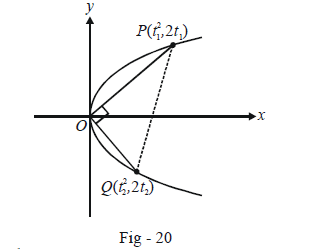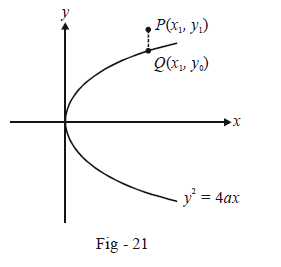# Basic Examples on Parabolas Set 2

Go back to  'Parabola'

Learn from the best math teachers and top your exams

• Live one on one classroom and doubt clearing
• Practice worksheets in and after class for conceptual clarity
• Personalized curriculum to keep up with school

Example – 9

Through the vertex O of a parabola $${y^2} = 4x,$$ chords OP and OQ are drawn at right angles to one another. Show that for all positions of P, PQ cuts the axis of the parabola at a fixed point. Also find the locus of the middle point of PQ.

Solution: Let P and Q be the points t1 and t2 so that their co-ordinates are $$(t_1^2,\,\,2{t_1}){\text{ }}and{\text{ }}(t_2^2,\,\,2{t_2})$$ respectively.Since $$PO \bot OQ,$$ we have

\begin{align}&\qquad \;\frac{{2{t_1} - 0}}{{t_1^2 - 0}} \times \frac{{2{t_2} - 0}}{{t_2^2 - 0}} = - 1 \\\\& \Rightarrow \quad 4{t_1}{t_2} + t_1^2t_2^2 = 0 \\\\&\Rightarrow \quad {t_1}{t_2}({t_1}{t_2} + 4) = 0\\\\&\Rightarrow \quad {t_1}{t_2} = - 4\qquad\qquad\qquad(\text{}since\,{\text{ }}both\,{t_1}\,and\,{t_2}\,are\,{\text{ }}non - zero) \end{align}

The equation of the chord PQ is

\begin{align} &\qquad \;\; PQ: \frac{{y - 2{t_1}}}{{x - t_1^2}} = \frac{{2{t_1} - 2{t_2}}}{{t_1^2 - t_2^2}} \\\\& \Rightarrow \quad ({t_1} + {t_2})(y - 2{t_1}) = 2(x - t_1^2) \\\\ &\Rightarrow \quad ({t_1} + {t_2})y - 2t_1^2 - 2{t_1}{t_2} = 2x - 2t_1^2 \\\\& \Rightarrow \quad 2x - ({t_1} + {t_2})y + 2{t_1}{t_2} = 0 \\\\ &\Rightarrow \quad 2x - ({t_1} + {t_2})y - 8 = 0\qquad\qquad\qquad( \text{since}\,{t_1}\,{t_2} = {\text{ }}-4) \end{align}

It is evident that this line always passes through (4, 0), whatever the value of $${t_1}\left( {\;and\;{\rm{ }}hence\;{\rm{ }}{t_2}} \right)$$ may be.

The mid-point M(h, k) of PQ is given by

$h = \frac{{t_1^2 + t_2^2}}{2},\,\,k = \frac{{2{t_1} + 2{t_2}}}{2}$

$${t_1}\;and\;{\rm{ }}{t_2}$$ can easily be eliminated from these relations using $${t_1}{t_2} = {\rm{ }} -4$$, to obtain

${k^2} = 2h - 8$

The locus of M is therefore

${y^2} = 2(x - 4)$

Example - 10

Let PQ be a variable focal chord of length $$l$$ of the parabola $${y^2} = 4ax.$$ From the vertex of this parabola, a perpendicular is dropped into this focal chord; d is the length of this perpendicular. Prove that $$l{d^2} = 4{a^3}.$$

Solution Let P be the point t so that Q is -\begin{align} \frac{1}{t},\end{align} since PQ is the focal chord. We have

$PQ = l = \sqrt {\left( {a{t^2} - \frac{a}{{{t^2}}}} \right) + {{\left( {2at + \frac{{2a}}{t}} \right)}^2}} \\\qquad \qquad \qquad \qquad \;\; = a{\left( {t + \frac{1}{t}} \right)^2}\begin{array}{*{20}{l}} {{\rm{}}}\\ {{\rm{}}\left( {{\rm{as\,in\,Example }}{\rm{ }}{\rm{ }} - 7} \right) \qquad \dots \left( 1 \right){\rm{}}} \end{array}$

The equation of PQ is

\begin{align}&\qquad \quad \frac{{y - 2at}}{{x - a{t^2}}} = \frac{{\begin{align}y + \frac{{2a}}{t}\end{align}}}{{\begin{align}x - \frac{a}{{{t^2}}}\end{align}}}\\&\Rightarrow \qquad y\left( {t - \frac{1}{t}} \right) = 2x - 2a\qquad\qquad\qquad...\left( 2 \right)\end{align}

The length of the perpendicular form the vertex, which is (0, 0), to PQ is,

\begin{align}& \qquad \;\ d = \frac{{2a}}{{\sqrt {4 + {{\left( {\begin{align}t - \frac{1}{t}\end{align}} \right)}^2}} }}\\&\Rightarrow \qquad {d^2} = \frac{{4{a^2}}}{{{{\left( {\begin{align}t + \frac{1}{t}\end{align}}\right)}^2}}}\qquad\qquad\qquad...\left( 3 \right) \end{align}

From (1) and (3), we have $$l{d^2} = 4{a^3}.$$

Example - 11

$$P({x_1},\,\,{y_1})$$ is a fixed point in the plane. How can we determine whether P lies inside or outside the parabola $${y^2} = 4ax\,\,?$$

Solution: Let us consider the case when a > 0. Then the parabola will open towards the right.

Consider the point $$P({x_1},\,\,{y_1})$$ lying in the plane as shown below :Since Q lies on the parabola, $$y_0^2 = 4a{x_1}$$

Since $${y_1} > {y_0},$$ we have $$y_1^2 > 4a{x_1}{\text{ }}or{\text { }}y_1^2 - 4a{x_1} > 0;$$ similarly, if P were to lie below the parabola, the same condition would be satisfied.

If P were to lie in the left half-plane, this condition would automatically be satisfied since x would be negative. Thus, we can now state the relative position of the point $$P\left( {{x_1},{\rm{ }}{y_1}} \right)$$ in terms of the equation $$S(x,y) = {y^2} - 4ax$$ as follows :

$$S({x_1},{y_1}) > 0 \qquad\Rightarrow \qquad P$$ lies outside the parabola

$$S({x_1},{y_1}) = 0 \qquad\Rightarrow\qquad P$$ lies on the parabola

$$S({x_1},{y_1}) < 0\qquad \Rightarrow \qquad P$$ lies inside the parabola.

Verify that these conditions would hold true if a < 0 too.

Parabolas
grade 11 | Questions Set 1
Parabolas
Parabolas
grade 11 | Questions Set 2
Parabolas